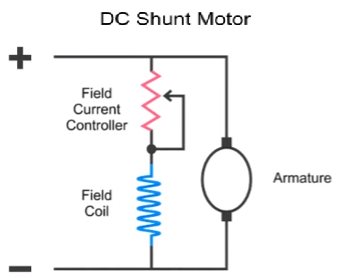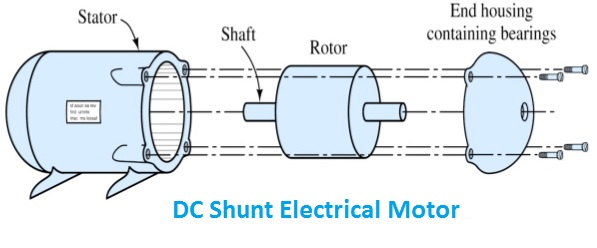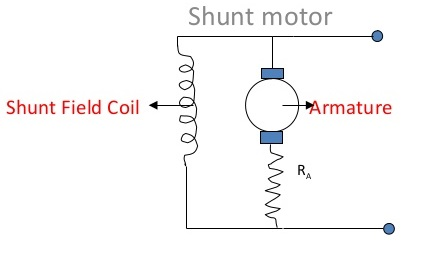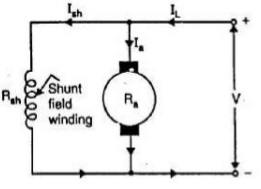# DC Shunt Motor – Definition & Principle

## What is a DC Shunt Motor?

The shunt wound DC motor falls under the category of self excited DC motors, where the field windings are shunted to, or are connected in parallel to the armature winding of the motor, as its name is suggestive of.

And for this reason both the armature winding and the field winding are exposed to the same supply voltage, though there are separate branches for the flow of armature current and the field current as shown in the figure of DC shunt motor below.### Construction of DC Shunt Motors

As you know that the construction of any type of DC Motor is very similar to a DC generator. This is also well known that the DC machines can be used as a generator as well as as a motor, their is the difference of operation in both case i.e in the case of motor the input to the machine is electrical power and the output is mechanical power and in the case of generator input is mechanical power and output is electrical power. Shunt Motors are special types of motors in which armature windings are connected in parallel.### Working Principle & Formula of DC Shunt Motors

For a DC shunt motor field winding is connected in series with the armature and current passing through the series winding is same as the armature current therefore the series field winding has fewer turns of thick wire than the shunt field winding. Due to this arrangement field winding will posses a low resistance then the armature winding.

As we can understand from the illustrations given the shunt field winding in a DC shunt motor has more number of turns with less cross-sectional area. Now let us understand the formula and example of DC shunt principle:Rsh is the field winding resistance.

Ra is the armature resistance. The value of armature resistance is very small.

The input voltage V is equal to the voltage across the armature and field winding.

IL is the line current drawn from the supply. The line Current is divided into two paths, one through the field winding and other through the armature.

IL = Ia + Ish

where, Ia = Armature current

Ish = Shunt field current

Ish = V / Rsh

In shunt motor, flux produced by field winding is proportional to the field current Ish. i.e., φ α Ish. Since the input voltage is constant, the flux generated is also constant. Therefore, DC shunt motor is called a constant speed motor as well.

The DC shunt motor torque is directly proportional to flux and armature current.

T α φ Ia

Φ is constant, therefore

T α Ia

#### Applications of DC Shunt Motors

DC shunt motors are used in:

• Blowers and fans
• Centrifugal and reciprocating pumps
• Lathe machines
• Machine tools
• Milling machines
• Drilling machines
##### Video Tutorial About DC Shunt Motors

error: Content is protected !!